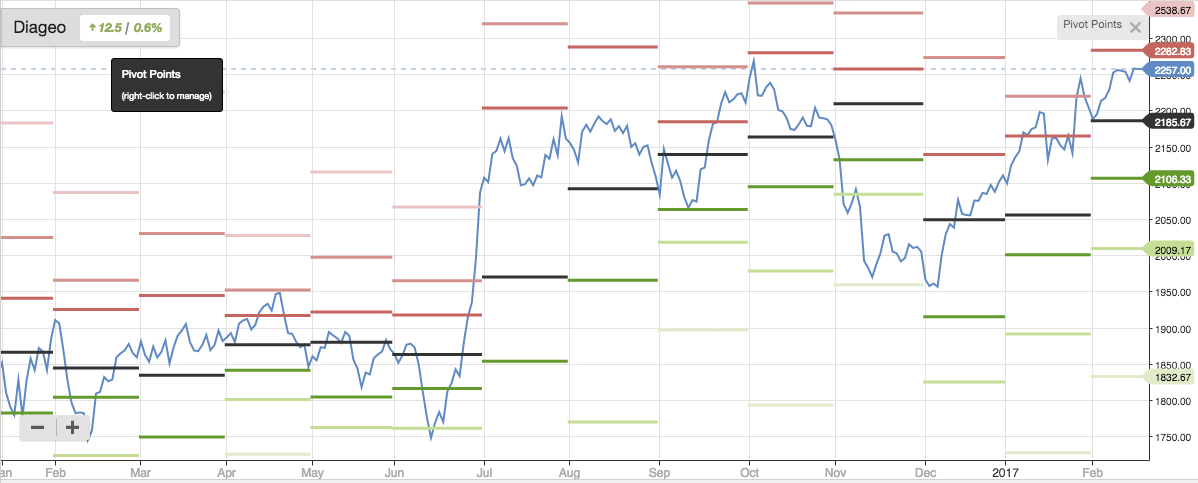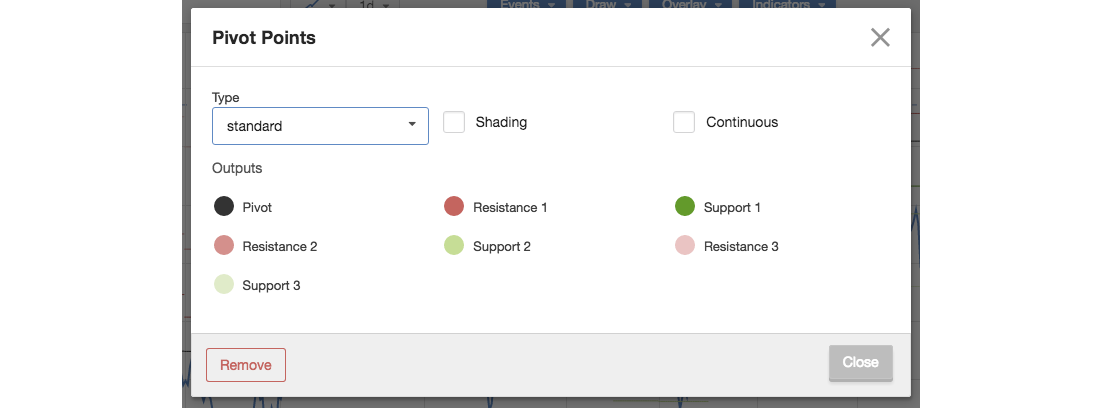# Pivot Points

Pivot Points are horizontal lines that plot the average of a company’s most recent high, low and closing price. They also overlay a series of support and resistance levels.

Typically, the top red lines represent resistance, while the bottom green lines represent support. The black line in the middle is the Pivot Point.

There are three pairs of support and resistance levels used in a Pivot Point overlay. Here are the calculations:

• Pivot point (P) = (H + L + C) / 3
• Third resistance level (R3) = P + 2 x (H – L)
• Second resistance level (R2) = P + (H – L)
• First resistance level (R1) = (2 x P) – L
• First support level (S1) = (2 x P) – H
• Second support level (S2) = P – (H – L)
• Third support level (S3) = P – 2 x (H-L) (H = Previous High, L = Previous Low, C = Previous Close)The main inputs for Pivot Points can’t be customised, but the colours used on the chart can be using the Pivot Point settings modal.